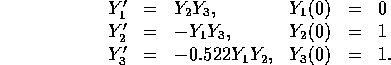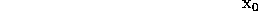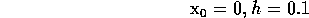Runge-Kutta MethodNext: Run Time Output Up: C-XSC Sample Programs Previous: Run Time Output

## Runge-Kutta Method

The initial value problem for a system of differential equations is to be solved by the well-known Runge-Kutta method. The C-XSC program is very similar to the mathematical notation. Dynamic vectors are used to make the program independent of the size of the system of differential equations to be solved.

Consider the first-order system of differential equationsIf the solution Y is known at a point x, the approximation Y(x+h) may be determined by the Runge-Kutta method:For example, we solve the systemThe program computes an approximation of the solution at the pointsstarting at given(here:).

```#include "rvector.hpp"

rvector F(real x, rvector Y)
{ // Function definition
rvector Z(3);               // Constructor call

x    =  0.0;                // F is independent of x
Z =  Y  * Y;
Z = -Y  * Y;
Z = -0.522 * Y * Y;
return Z;
}

void Init(real& x, real& h, rvector& Y)
{                             // Initialization
Resize(Y,3);                // Resize dynamic array
x    = 0.0;   h    = 0.1;
Y = 0.0;   Y = 1.0;  Y = 1.0;
}

main()
{
real x, h;                  // Declarations and dynamic
rvector Y(3), K1(3), K2(3), K3(3), K4(3); // memory allocation

Init(x, h, Y);
for (int i=1; i<=3; i++) {  // 3 Runge-Kutta steps
K1 = h * F(x, Y);         // with array result
K2 = h * F(x + h / 2, Y + K1 / 2);
K3 = h * F(x + h / 2, Y + K2 / 2);
K4 = h * F(x + h, Y + K3);
Y  = Y + (K1 + 2 * K2 + 2 * K3 + K4) / 6;
x += h;
cout << SetPrecision(18,16) << Dec;     // I/O modification
cout << "Step:  " << i << ",  "
<< "x =    " << x << endl;
cout << "Y =    " << endl << Y << endl;
}
}```

Andreas Wiethoff
Wed Mar 29 13:50:24 MET DST 1995